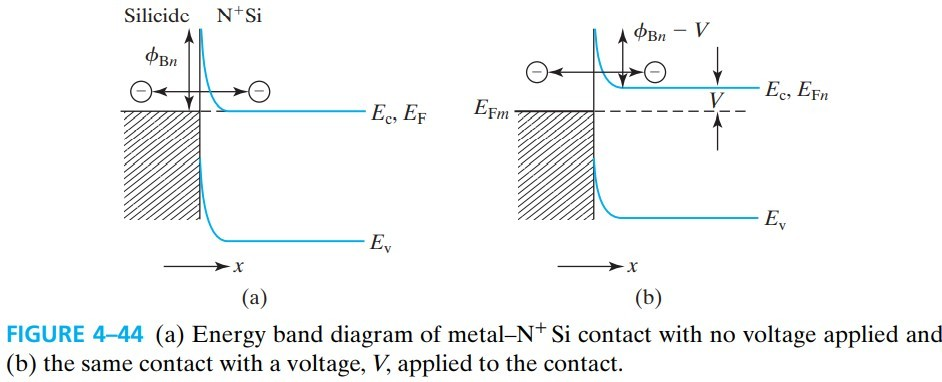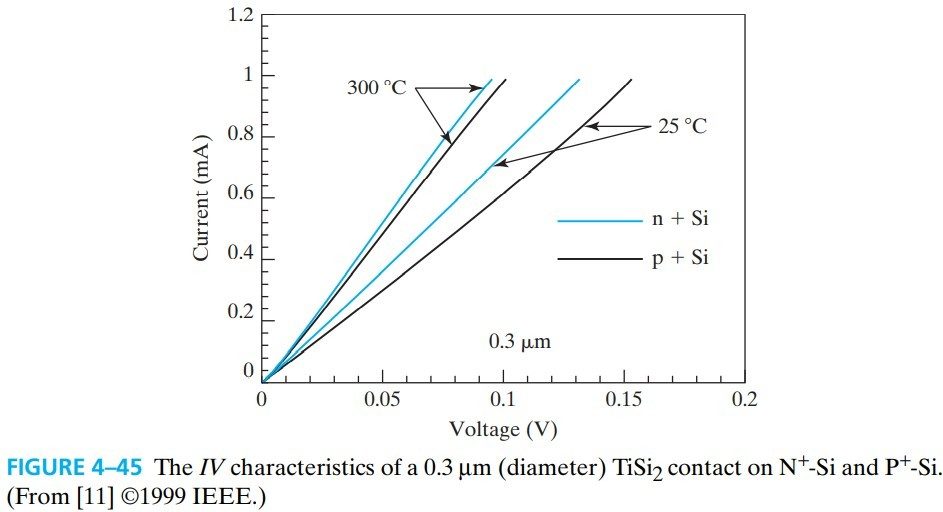# PN and Metal-Semiconductor Junctions

PN junction 就是 P-type 和 N-type 靠在一起，其主要特性是 rectifying current–voltage（见 fig 4-2），所以也叫 rectifier or diode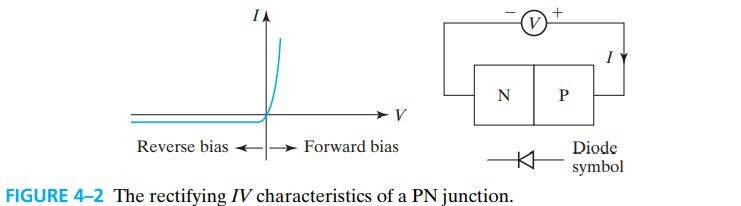# 4.1 Building Blocks of the PN Junction Theory

## 4.1.1 Energy Band Diagram and Depletion Layer of a PN Junction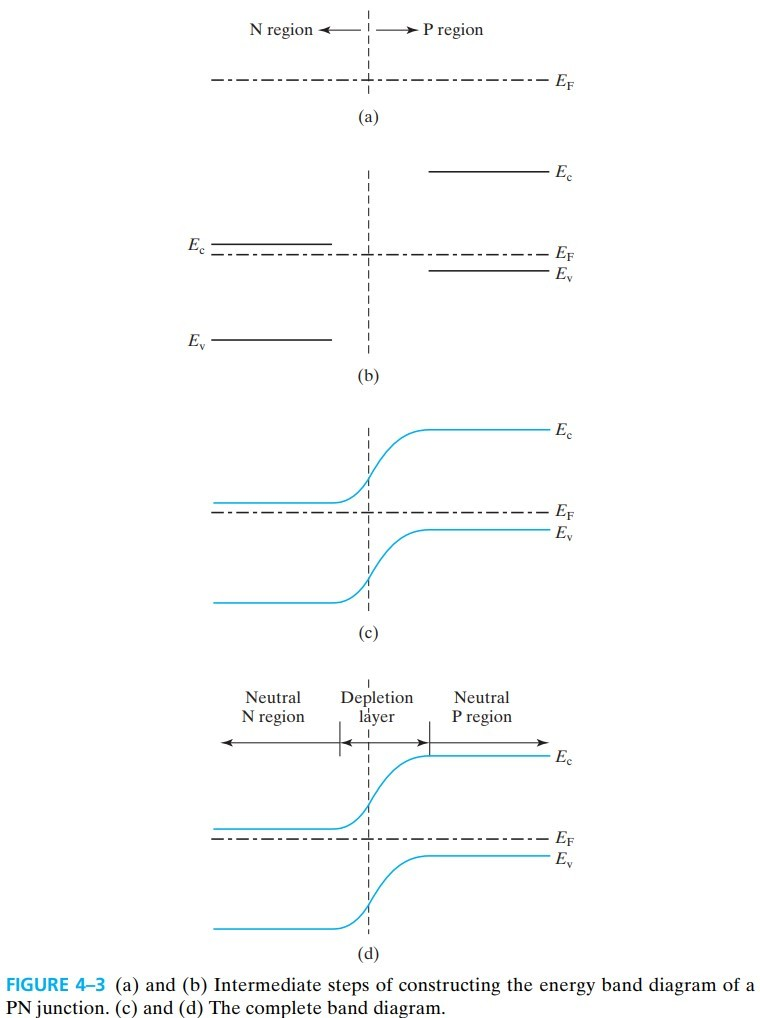• 左边：the neutral N layer
• 右边：the neutral P layer
• 中间：depletion layer 费米能级在中间，表示 $n\approx p \approx 0$

## 4.1.2 Built-In Potential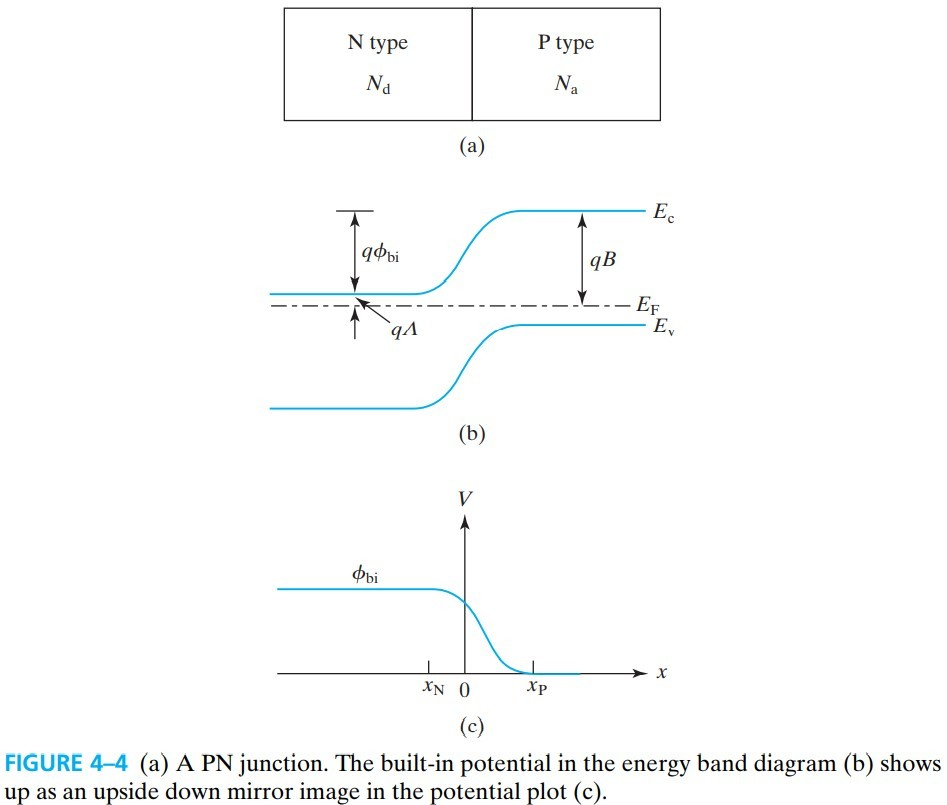## 4.1.3 Poisson’s Equation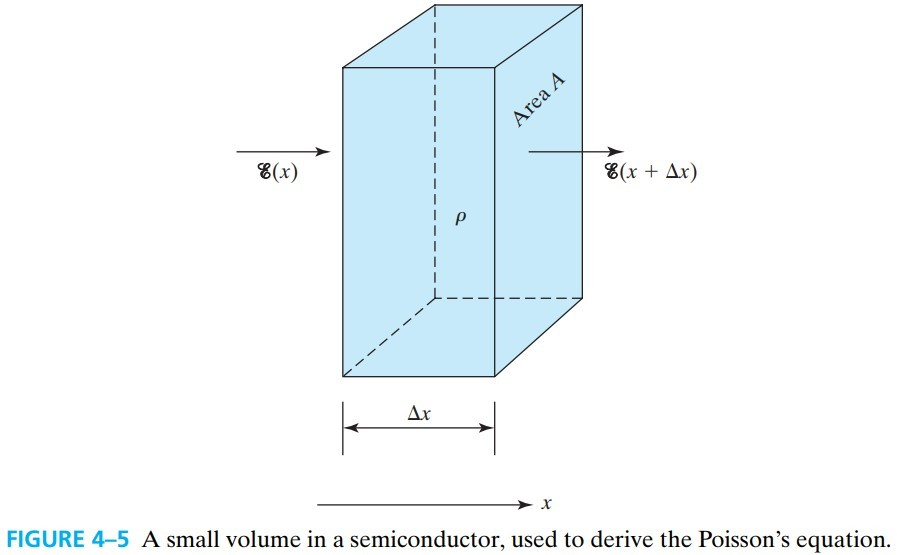## 4.2 Depletion-Layer Model

### 4.2.1 Field and Potential in the Depletion Layer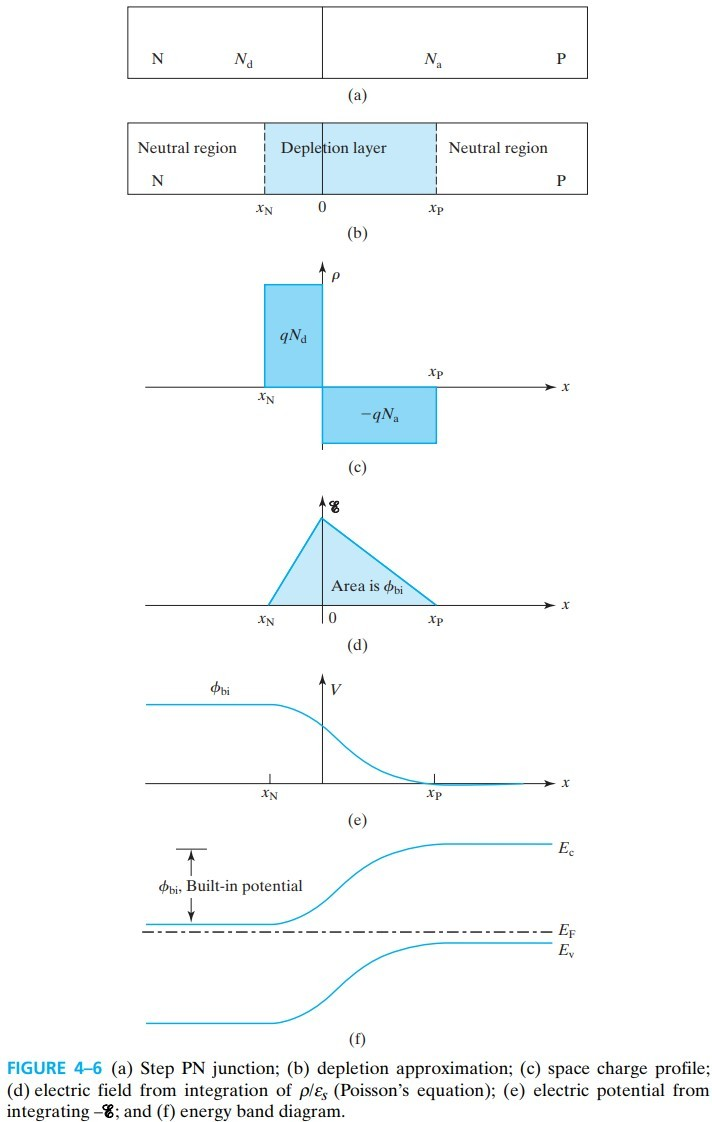## 4.2.2 Depletion-Layer Width

• If $N_a \gg N_d$, as in a ${\rm P^+N}$ junction, $W_\text{dep} \approx \sqrt{\frac{2 \varepsilon_s \phi_\text{bi}}{qN_d}} \approx |x_N| \tag{4.2.9}$
• If $N_d \gg N_a$, as in an $\rm{ N^+P}$ junction, $W_\text{dep} \approx \sqrt{\frac{2 \varepsilon_s \phi_\text{bi}}{qN_a}} \approx |x_P| \tag{4.2.10}$

# 4.4 Capacitance-Voltage Charateristics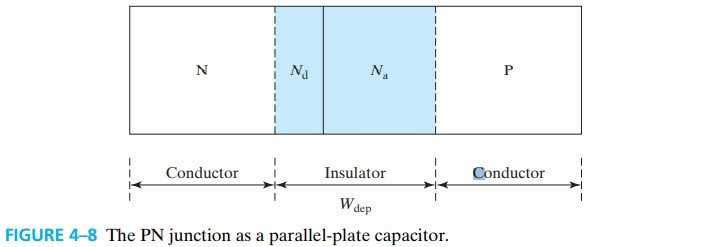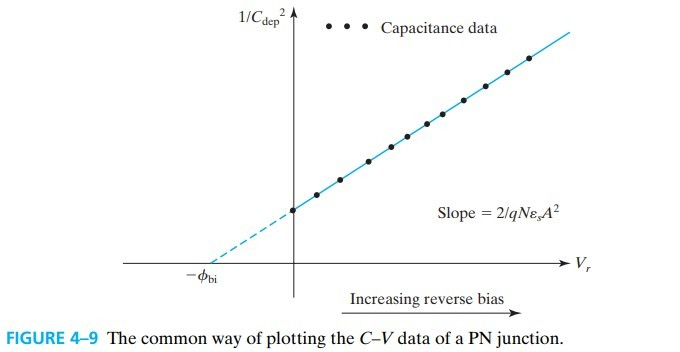# 4.5 Junction Breakdown

Junction Breakdown 指的是当反偏电压大于某个值时，电流急剧增大的情况。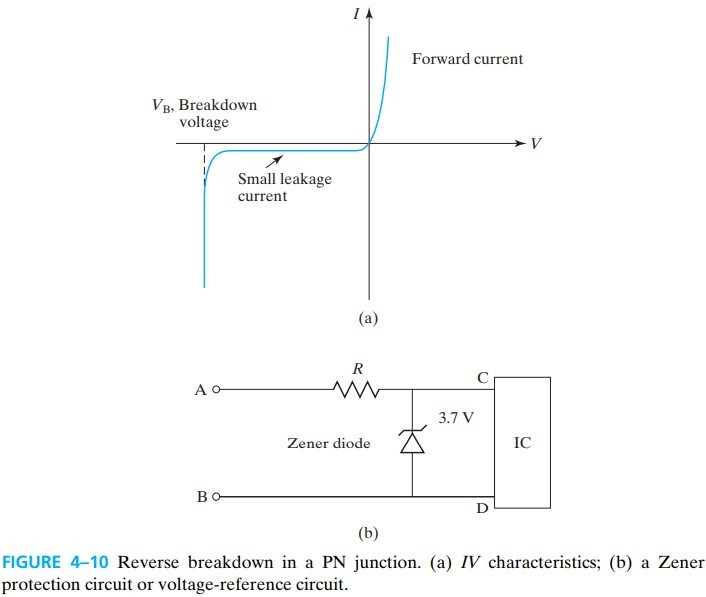## 4.5.1 Peak Electric Field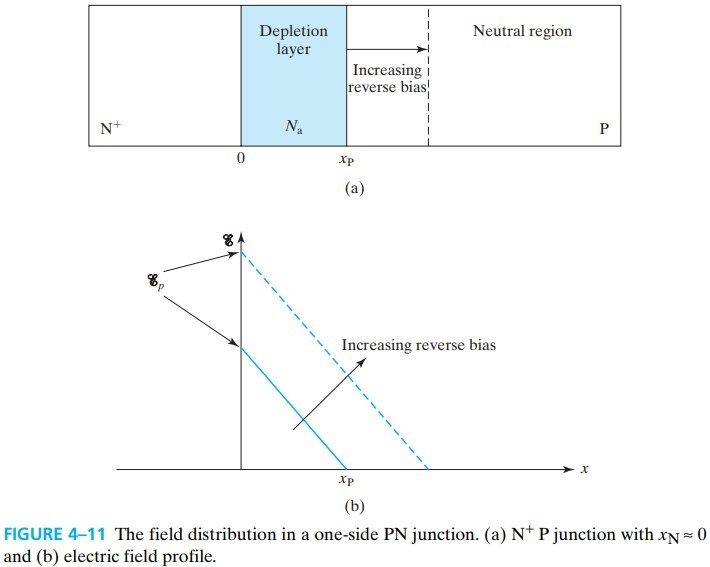## 4.5.2 Tunneling Breakdown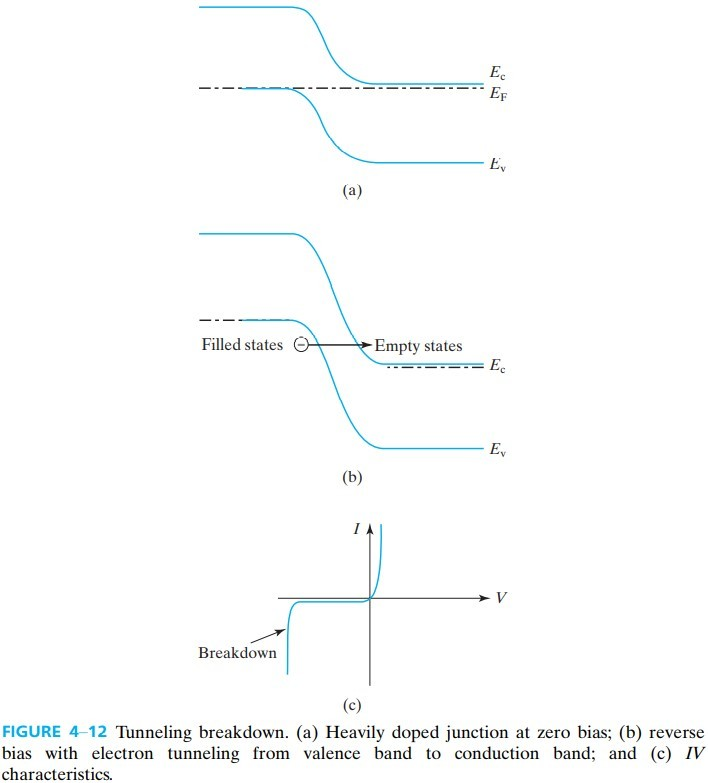tunneling current density：$J = G e^{-H/\mathscr{E}_p}$

## 4.5.3 Avalanche Breakdown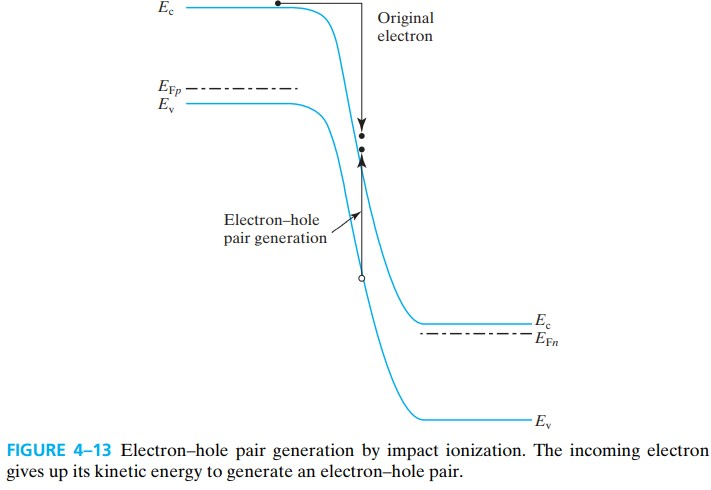# 4.6 Carrier Injection Under Forward Bias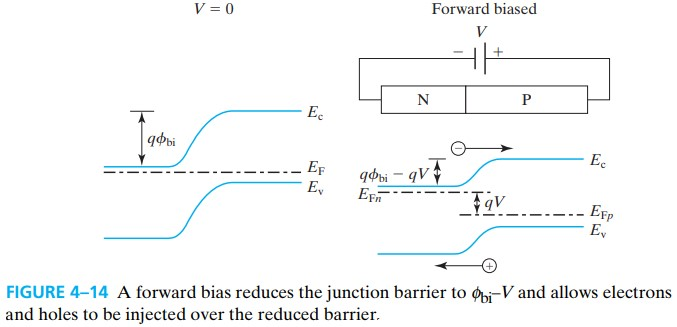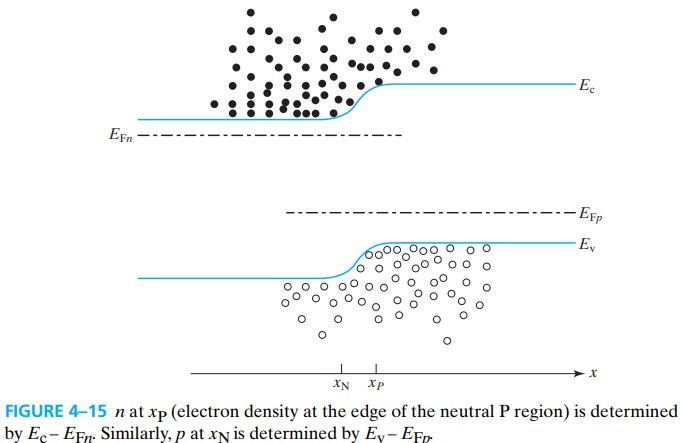# 4.7 Current Continuity Equation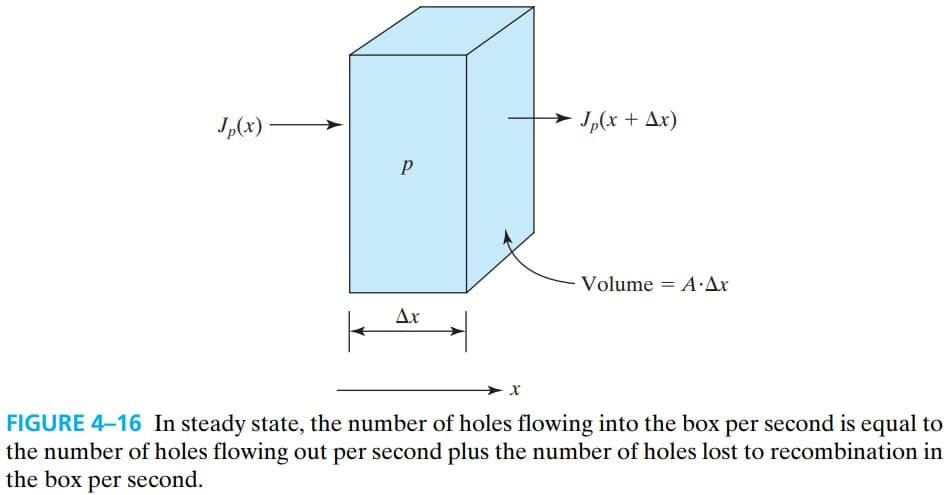$D_p$ 是空穴的扩散系数 diffusion constant. 由于 $p=p_0+p’$，$p_0$ 取决于 $N_a$，是不变的，所以微分部分可以减去常数 $p_0$，并进行变形：

$L_n$ 和 $L_p$ 称为电子和空穴的 diffusion lengths

# 4.8 Excess Carriers in Forward-Biased PN Juntion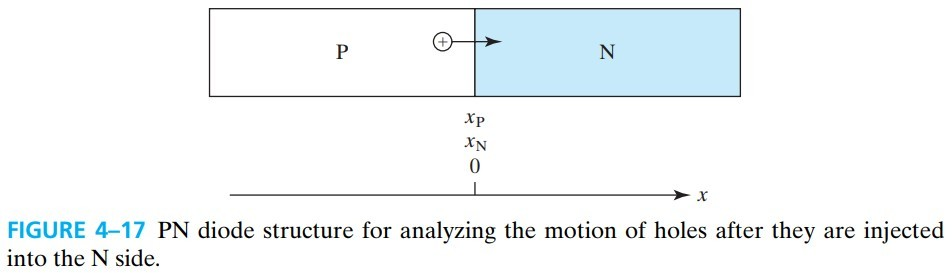$x_N,x_P$ 和 $L_n,L_p$ 带来的影响比较小（一般都是同一数量级），主要看 $p_{N0}$ 和 $n_{P0}$，由于掺杂越少，少子就越多，因此可以得出：轻掺杂一侧的少子注入更多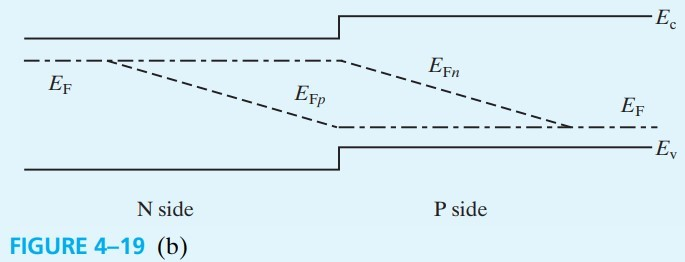# 4.9 PN Diode I-V Characteristics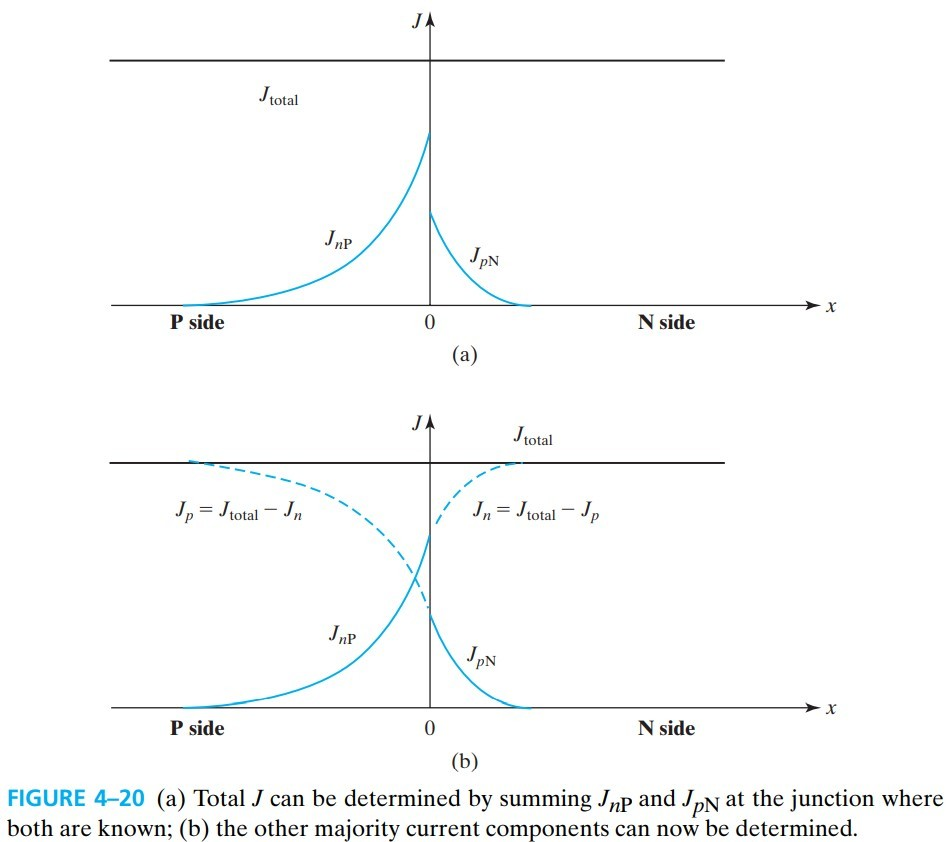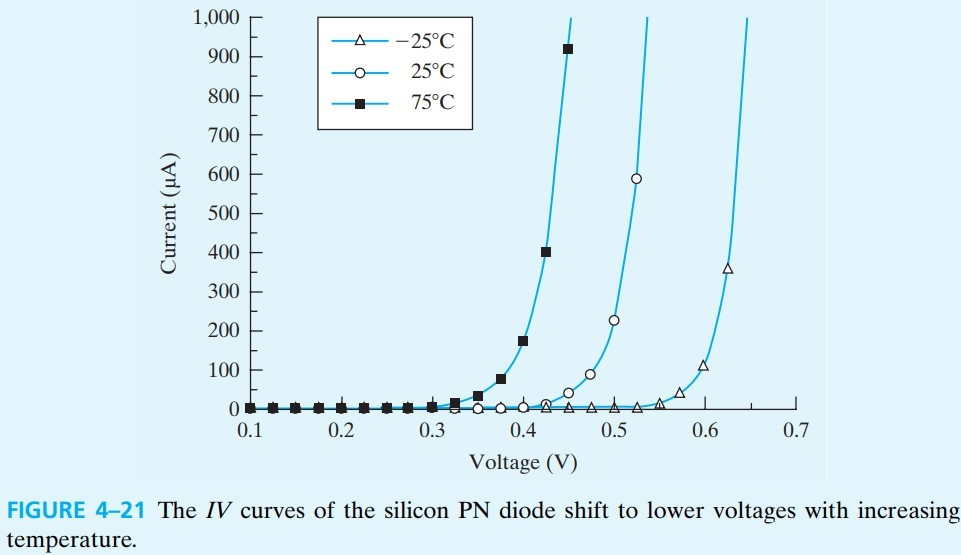## 4.9.1 Contributions from the Depletion Region

$\tau_\text{dep}$ is the generation/recombination lifetime in the depletion layer.（不太清楚这是啥，总之题目会给吧），后面减 1 是为了在没有偏压时，净复合率为 0.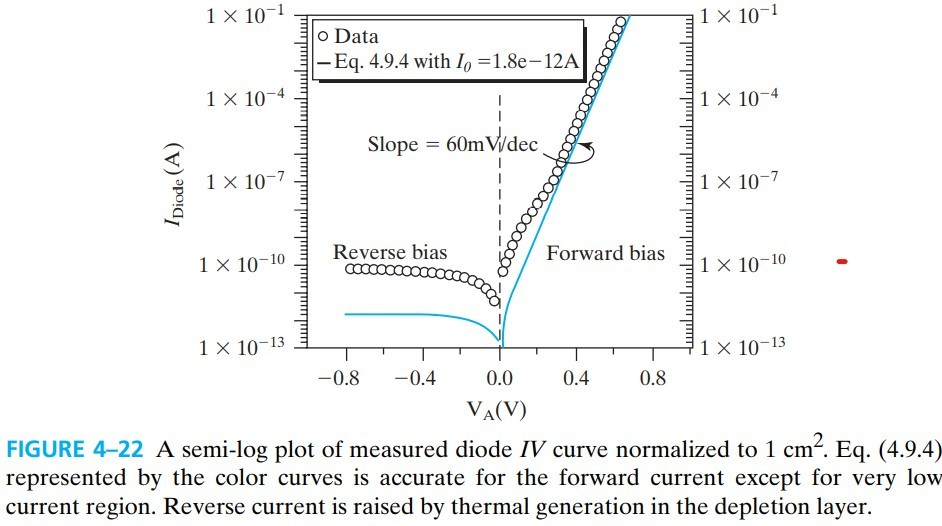# 4.10 Charge Storage

$\tau_s$ is called the charge-storage time. In a one-sided junction, $\tau_s$ is the recombination lifetime on the lighter-doping side, where charge injection and recombination take place.

# 4.11 Small-Signal Model of the Diode

diode 在高频小信号电路中等效为 RC 并联：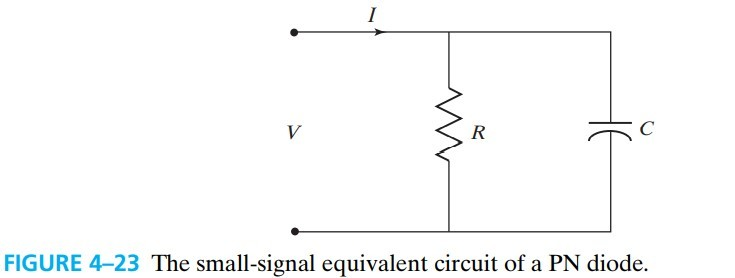# 4.16 Schottky Barriers

two kinds of metal–semiconductor junction

• Schottky diodes： metal and lightly doped semiconductors
• ohmic contacts：metal and heavily doped semiconductors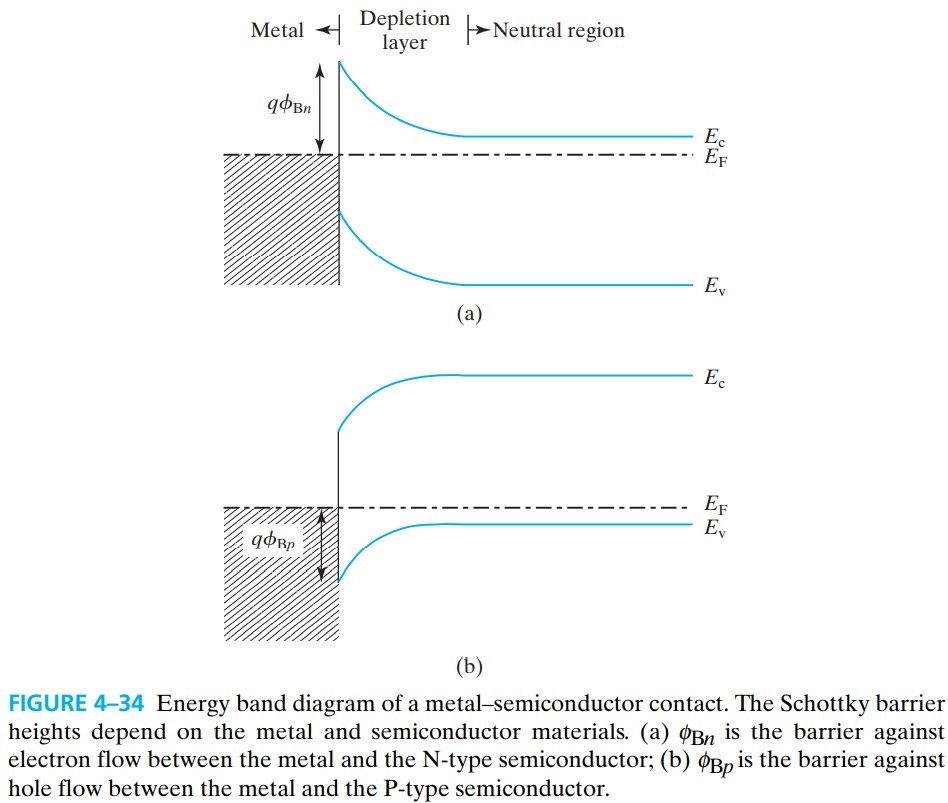$\phi_B$ 称为 Schottky barrier height，常见金属的肖特基势垒如下表：

Metal Mg Ti Cr W Mo Pd Au Pt
$\phi_{Bn}$（V） 0.4 0.5 0.61 0.67 0.68 0.77 0.8 0.9
$\phi_{Bp}$（V）   0.61 0.50   0.42   0.3
$\psi_M$（V） 3.7 4.3 4.5 4.6 4.6 5.1 5.1 5.7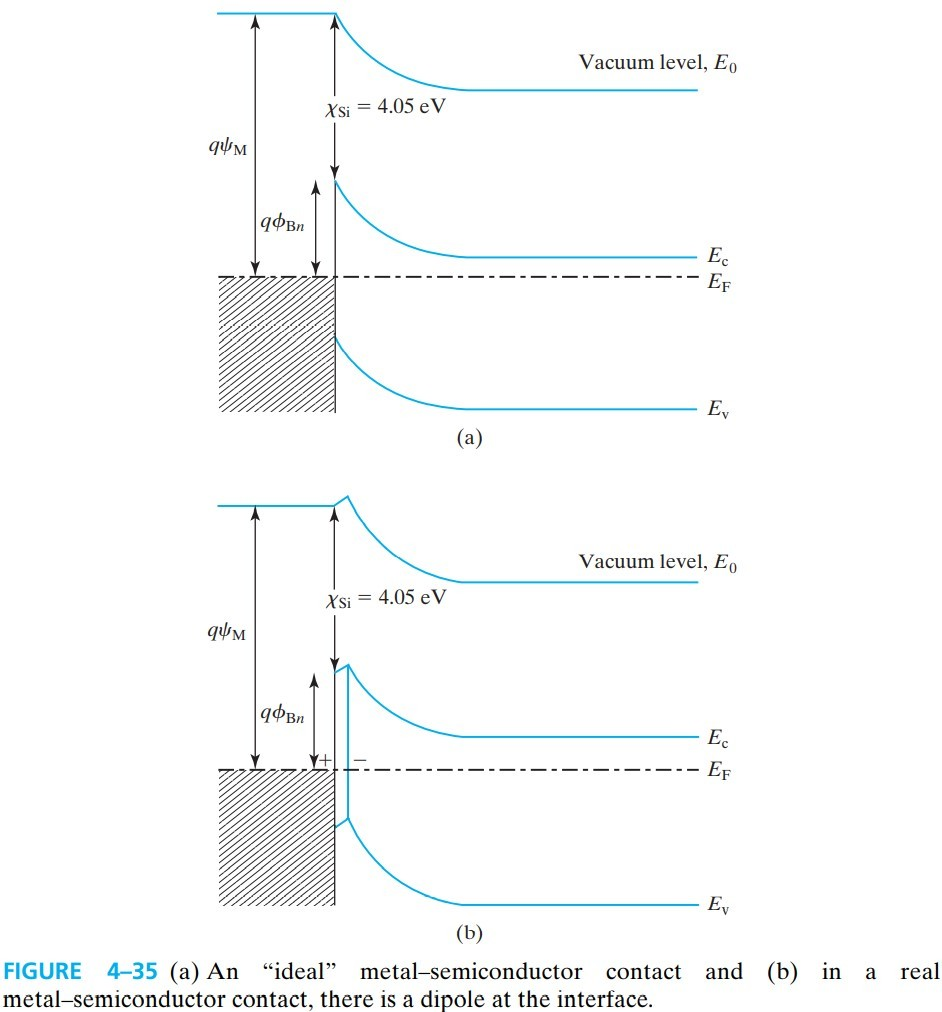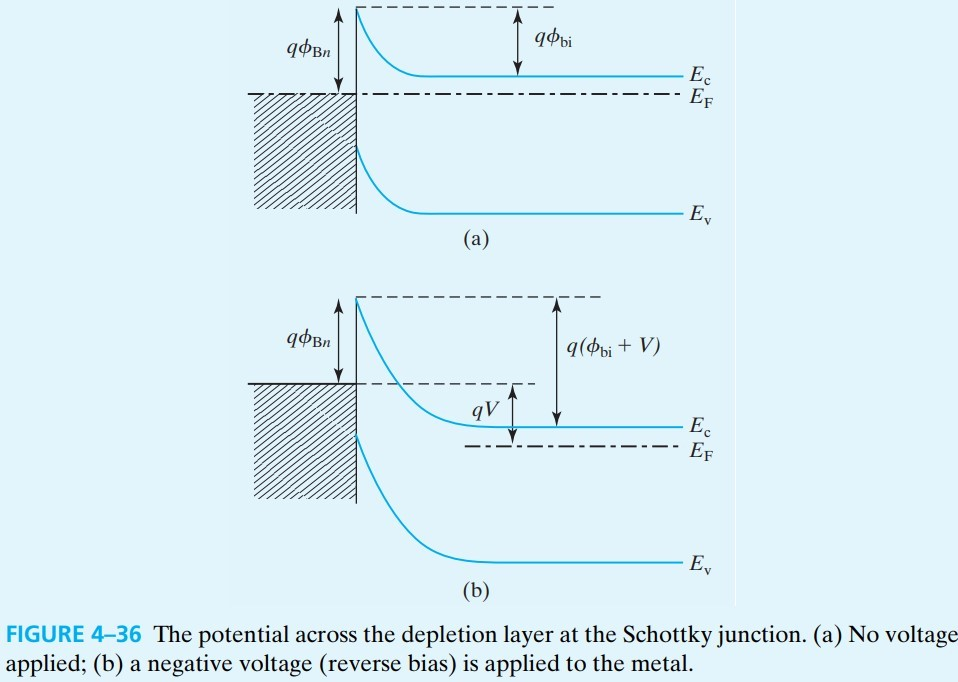# 4.17 Thermionic Emission Theory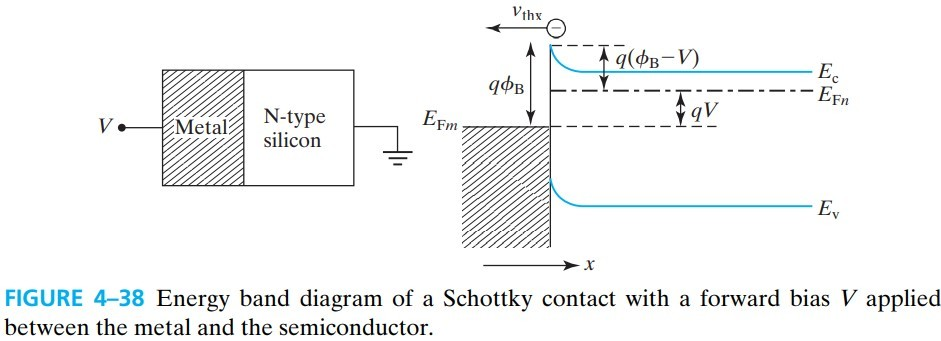$K\approx 100 {\rm A/(cm^2/K)}$ 称为 Richardson constant

# 4.18 Schottky Diodes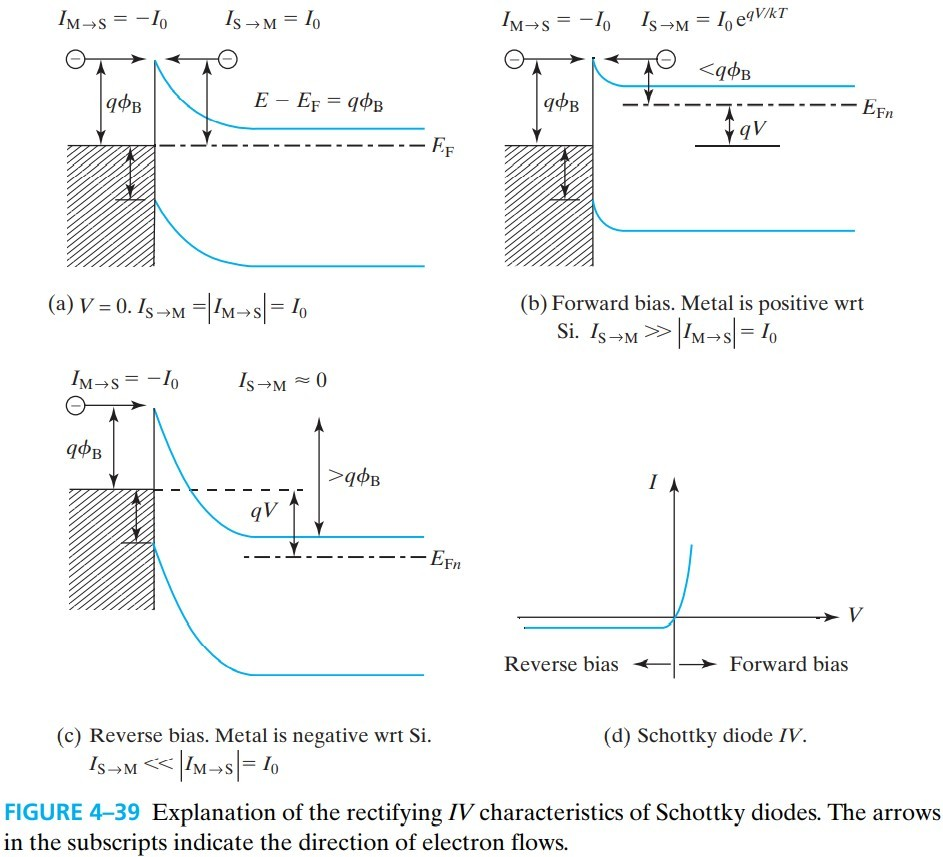# 4.20 Quantum Mechanical Tunneling

$m$ is the effective mass and $h$ is the Planck’s constant.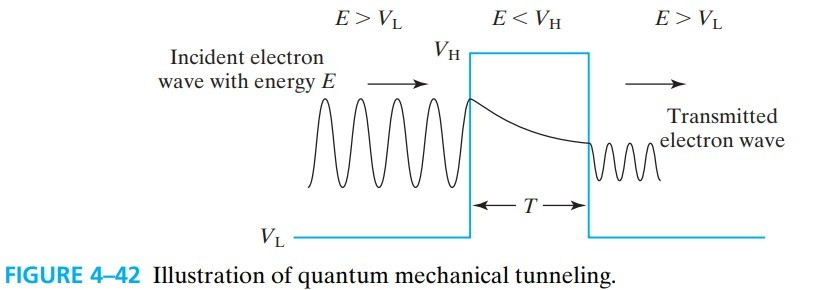# 4.21 Ohmic Contacts